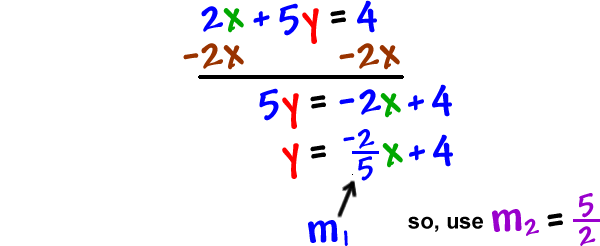Check it out:Take the 2 ... Write it as a fraction: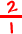Flip it over:... and change the sign: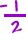Let's do a problem:

 Let's find the equation of the line that passes through the point ( 1 , 3 ) and is perpendicular to the line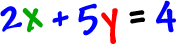.

What do we need?

 a point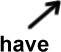a slopeSTEP 1:  Find the slope

 It's perpendicular to...

So, we'll get this guy's slope ...  flip it and change the sign!  Easy!